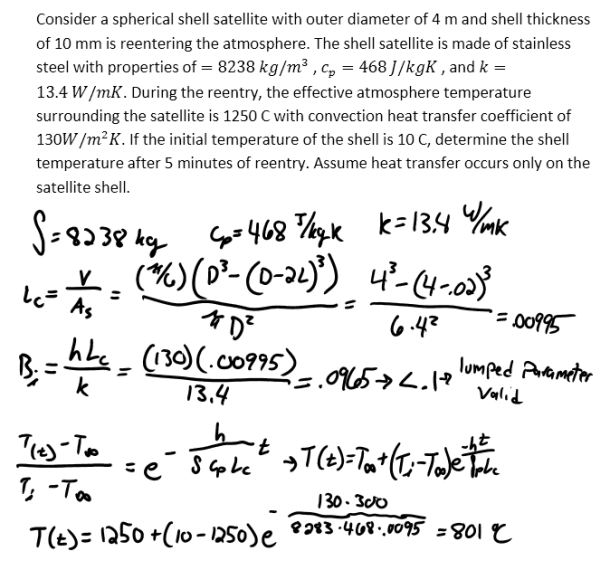heat and mass transfer problems engineering equations heat and mass transfer school homework engineering heat and mass transfer formulas heat and mass transfer solutions to heat and mass transfer problems full solution engineering problem solution heat and mass transfer math problems engineering equations heat and mass transfer school homework engineering solutions to heat and mass transfer formulas heat problems mass problem solutions to transfer problems full solution heat and mass transfer
heat and mass transfer problems engineering equations heat and mass transfer school homework engineering heat and mass transfer formulas heat and mass transfer solutions to heat and mass transfer problems full solution engineering problem solution heat and mass transfer math problems engineering equations heat and mass transfer school homework engineering solutions to heat and mass transfer formulas heat problems mass problem solutions to transfer problems full solution heat and mass transfer
Highalphabet Home Page heat and mass transfer problem solutions Heat and Mass Transfer Page
Consider a spherical shell satellite with outer diameter of 4 m and shell thickness of 10 mm is reentering the atmosphere. The shell satellite is made of stainless steel with properties of =8238 kg/m^3 , c_p=468 J/kgK , and k=13.4 W/mK. During the reentry, the effective atmosphere temperature surrounding the satellite is 1250 C with convection heat transfer coefficient of 130W/m^2 K. If the initial temperature of the shell is 10 C, determine the shell temperature after 5 minutes of reentry. Assume heat transfer occurs only on the satellite shell.Consider a spherical shell satellite with outer diameter of 4 m and shell thickness of 10 mm is reentering the atmosphere. The shell satellite is made of stainless steel with properties of =8238 kg/m^3 , c_p=468 J/kgK , and k=13.4 W/mK. During the reentry, the effective atmosphere temperature surrounding the satellite is 1250 C with convection heat transfer coefficient of 130W/m^2 K. If the initial temperature of the shell is 10 C, determine the shell temperature after 5 minutes of reentry. Assume heat transfer occurs only on the satellite shell.